# Combine Mathematical Approaches with Native Text-Based Math in LabVIEW

Publish Date: Jun 06, 2013 | 1 Ratings | 5.00 out of 5 | Print | Submit your review

## Overview

In LabVIEW, you have the freedom to choose the syntax you prefer for analysis when developing algorithms, analyzing results, or processing signals. Although LabVIEW is well-known as a development environment for a graphical programming language, it also offers math-oriented textual programming through a native compiler for .m files. This compiler, LabVIEW MathScript, uses the .m file script syntax and includes more than 800 commonly used functions for math, signal processing, analysis, and control. The LabVIEW MathScript RT Module is an add-on for LabVIEW that installs the LabVIEW MathScript compiler along with two interfaces to implement your custom .m files.

#### Interactively Explore Your Data with the MathScript Window

The LabVIEW MathScript Window offers an interactive interface in which you can load, save, design, and execute your .m files. It is designed for concept exploration through a command-line interface that you use to enter commands one at a time or through the building of batch scripts in a simple text editor window. Figure 1 displays the LabVIEW MathScript Window, which you can access from the LabVIEW menus by choosing Tools»MathScript Window.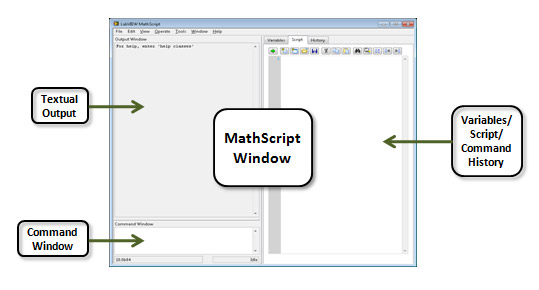##### Figure 1. LabVIEW MathScript Window for Interacting with Your Custom .m Files

The LabVIEW MathScript Window provides immediate feedback in a variety of formats including graphs and text. You can use a variety of plotting commands to generate graphs from the LabVIEW MathScript Window similar to Figure 2.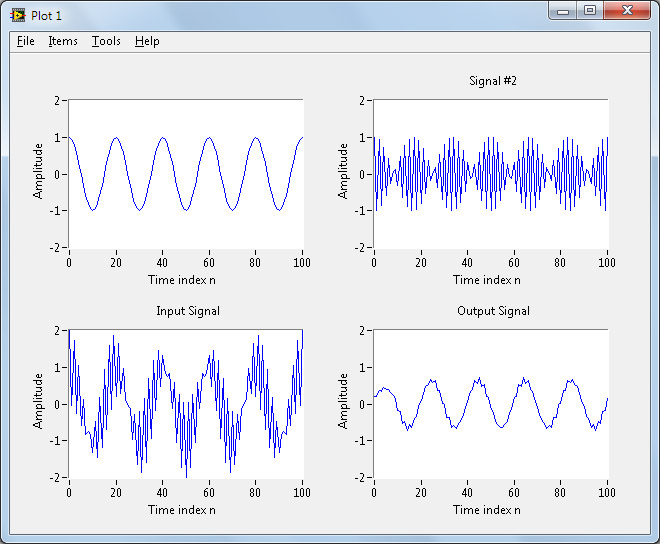##### Figure 2. Example Plot Window Generated from LabVIEW MathScript

You can generate a wide variety of plots from MathScript including the following:

• Bar (2D and 3D)
• Contour (2D and 3D)
• Errorbar
• Feather
• Mesh
• Pie
• Polar
• Scatter
• Surface
• Tree
• Waterfall

View for more information on using the MathScript Interactive Window for algorithm development

#### Embed Your Custom .m File with LabVIEW Graphical Code and the MathScript Node

Combining textual programming with traditional LabVIEW graphical programming is also possible using a script node interface. Script nodes are resizable text entry regions on the LabVIEW block diagram that you can add to your graphical programs. Through the MathScript Node, you can execute scripts during the run-time execution of a VI. Data enters the left border of the node, is used or modified during the sequential execution of the script text, and then exits the node through an output variable on the right border of the node.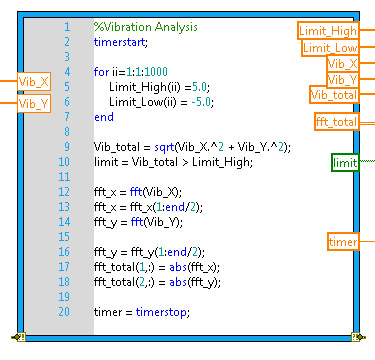##### Figure 3. MathScript Node Places Your Custom .m File Code Inline with Graphical G Code

You can type your scripts, copy and paste, or import them from a file. With the MathScript Node, you can reuse your custom .m files, even if you developed them outside LabVIEW MathScript, to bring your text-based math routines inline with your data acquisition in the graphical LabVIEW environment.

With LabVIEW MathScript and LabVIEW graphical programming, you have the power to choose the most appropriate syntax, which can often be a combination of the two. Consider the following script from the widely used textbook Digital Signal Processing Laboratory Using MATLAB® by Sanjit Mitra. It generates a test signal and then applies a moving-average filter to it.

% Simulation of an M-point Moving Average Filter
% Generate the input signal
n = 0:100;
s1 = cos(2*pi*0.05*n); % A low-frequency sinusoid
s2 = cos(2*pi*0.47*n); % A high frequency sinusoid
x = s1+s2;
% Implementation of the moving average filter
M = input('Desired length of the filter = ');
num = ones(1,M);
y = filter(num,1,x)/M;
% Display the input and output signals
subplot(2,2,1);
plot(n, s1);
axis([0, 100, -2, 2]);
xlabel('Time index n'); ylabel('Amplitude');
title('Signal #1');
subplot(2,2,2);
plot(n, s2);
axis([0, 100, -2, 2]);
xlabel('Time index n'); ylabel('Amplitude');
title('Signal #2');
subplot(2,2,3);
plot(n, x);
axis([0, 100, -2, 2]);
xlabel('Time index n'); ylabel('Amplitude');
title('Input Signal');
subplot(2,2,4);
plot(n, y);
axis([0, 100, -2, 2]);
xlabel('Time index n'); ylabel('Amplitude');
title('Output Signal');
axis;

This script generates two sinusoid signals, adds them together, and then applies a moving-average filter to the sum of the two. Figure 2 is the plot generated from this script. The LabVIEW MathScript Window provides an interface to interact with the script one run at a time. However, combining this script with the LabVIEW graphical programming paradigm provides a powerful method to automate the script, giving you the ability to interact with your input parameters on the fly.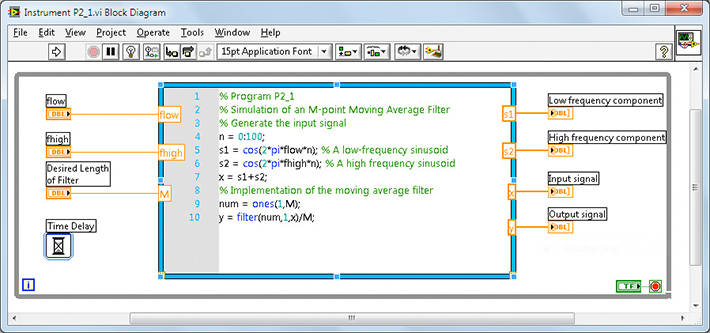Figure 4. Example Block Diagram Integrating Text-Based Math with G Code in LabVIEW

Figure 4 displays the integration of the script into the LabVIEW block diagram using the MathScript Node. Two changes were made to the script:

1. The input parameters flow, fhigh, and M are controlled using front panel controls.
2. The last 23 lines of the script were used to generate the plot. Those were removed, and the plots were configured using standard LabVIEW graphs.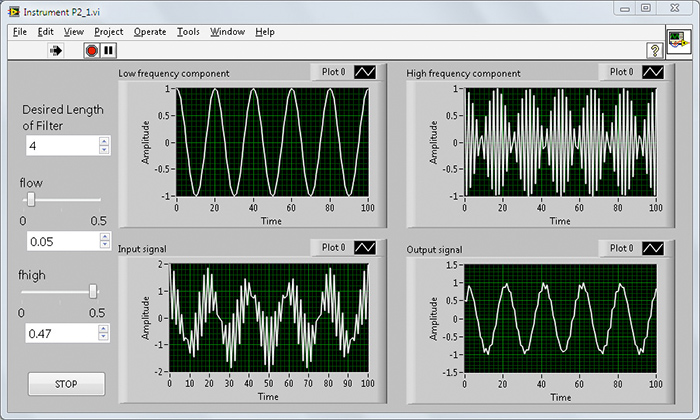Figure 5. Example Front Panel Integrating LabVIEW User Interface Components with Text-Based Math

With this LabVIEW front panel, you can control the low and high frequencies of the generated sinusoids as well as the length of the moving-average filter being implemented. As the LabVIEW VI runs, you can change these values and easily visualize the output of the analysis routine and how it is affected by the changes to the input values. This common procedure of applying the interactivity of a LabVIEW VI to a text-based script is known as instrumenting your algorithm.

### Ratings

Rate this document